Start typing, then use the up and down arrows to select an option from the list.## Organic Chemistry

Learn the toughest concepts covered in Organic Chemistry with step-by-step video tutorials and practice problems by world-class tutors.

1. A Review of General Chemistry

We use our knowledge of valance electrons to determine what he formal charge of the molecule will be.

Formal and Net Charge
1
concept

## Calculating formal and net charge.1m
Play a video:
So now that we understand bonding preferences so well, I want to move to a really related topic called formal charges. And formal charges are just based on the entire idea of bonding preferences. So let's go ahead and just jump right into it. Basically, a formal charges assigned whenever there's a difference between the number of Valence electrons and Adam wants toe have and the number of valence electrons it actually has. So remember that the group number is how maney it wants the valence electrons, the sticks and the dots are the it actually has. So all you do to calculate formal charge is you take the group number, whatever that is, that could be Group four, Group five, whatever. And then you subtract the valence electrons, which is just the sticks and the dots. Okay, It's really simple math. A lot of times, you'll just be able to do this on your fingers. All right, actually, all the time. So you take your group number, then you just subtract the sticks and the dots and you're good. All right. That's called the formal charge. The net charge. Let's write that down. The net charge is the term that we give for the sum of all the formal charges. Now, this is an important point because I remember when I was an undergrad. You see it a little bit confused over like, Oh, does the formal charge go on the whole thing? Or is it just one, Adam? No. The formal charges one atom at a time. You're just looking at each Adam and saying, Does this have a formal charge? Does this have a formal charge? The net charges the collection of all of those sums together. Okay, hopefully not too complicated. There's usually not that many formal charges on the molecule.

Easiest formal charge formula you will find anywhere on the internet:The Net Charge is the sum of all the formal charges on a molecule.

Calculate the formal charge of the following molecule: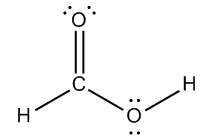2
concept

## Calculate the formal charges of ALL atoms.2m
Play a video:
Let's go ahead and do this example where I wanna look at each atom and I want to count the formal charges for all of them. What that means is I'm gonna be looking at group numbers and I'm gonna be looking at sticks and thoughts. So let's start off with the hydrogen even though we already know that this fits the bonding preference. Remember that Bonding preferences say that hydrogen wants toe. Have what? One stick, one bond. But let's just do it anyway. Hydrogen is in, What group? One. How many sticks does it have? One. How many thoughts does it have? Zero. So it's gonna be one according to the equation. My equation says that formal charge equals group number, which is one minus sticks and thoughts, which is one. So it's zero. So this has a zero formal charge. Do you see that? But it also fits with my bonding preference. So as long as you're bonding, preference agrees with what you see, that's going to be zero. Let's do this with this oxygen right here. Okay, So first of all, does this oxygen fit my bonding preference According Thio Bond represent, Yes, it does. It already fits it. So I could already put a zero here. Okay, but now I want to show you mathematically how it works out to. So we said that the formal charge equals the group number. What's the group? Number of oxygen. It's six minus the number of sticks and dots. So how maney is that All together? That's six. So then I would get a formal charge of zero, See how easy that is. So now you do that for carbon, but its carbon foot fulfilling its bonding preference. It already is. So I can just put a zero there. Okay, then finally, there was a type of just so you know, So notice that in your page the oxygen did not have those lone pairs. Go ahead and just write those in. Okay, that's just didn't copy. Okay, Um so basically, for this one on the oxygen has six electrons total. It wants six electrons. I'm sorry. And it has six electrons total in terms of the valence 66 sticks and dots. So should also be zero. This is another way that you can fulfill your bonding preference by having a double bond and having to lone pairs, it still counts is to Bonds and two lone pairs. So I hope that you guys see how, if it fits, it's bonding perfect. You don't need to calculate, but calculating is always just safe to do.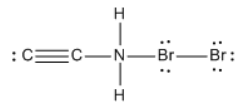3
Problem

Calculate the net charge of the molecule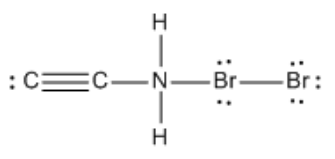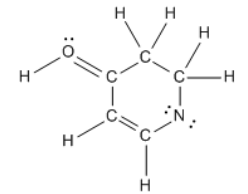4
Problem

Calculate the total charge of the molecule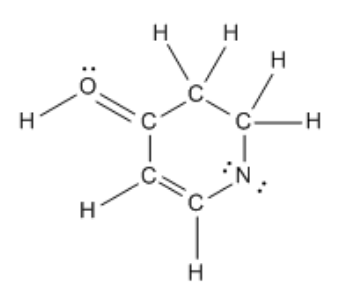Hell yeah! That’s literally all there is to know. Don’t let any professors complicate it more for you.